# NEET Important Chapter - Ray Optics and Optical Instruments

Get plus subscription and access unlimited live and recorded courses## Ray Optics and Optical Instruments - An Important Concept for NEET

Let us first understand what optics is. Optics is a branch of physics that deals with the behaviour of light or other electromagnetic waves. Our eyes have the ability to detect electromagnetic waves in a certain range of wavelengths. Using this ability, we gain knowledge of the whole world.

Light is a wave that travels in a straight light and doesn’t require a medium for its propagation. The path is called a ray of light and a bundle of such rays is called the beam of light.

In this chapter, students will learn about the behaviour of a beam of light when it encounters optical devices like mirrors and lenses. This part of optics is called geometric optics. Going further in this chapter, students will also learn about the construction and working of some important optical instruments and devices.

Now, let us move on to the important concepts and formulae related to the NEET exam along with a few solved examples.

### Important Topics of Ray Optics and Optical Instruments

• Reflection of Light

• Mirror Formula

• Refraction of Light

• Lens

• Total Internal Reflection

• Deviation by a Prism

• Dispersion by a Prism

• Optical Instruments

• Resolving Power of Optical Instruments

### Important Concepts of Ray Optics and Optical Instruments

 Name of the Concept Key Points of the Concept 1. Reflection of Light Reflection of Light is the phenomenon of bouncing back of light after striking a smooth surface. According to laws of reflection, the incident ray, reflected ray and the normal ray, all lie in the same plane.Also, during reflection, angle of incidence is equal to the angle of reflection. 2. Reflection from a spherical mirror Spherical mirrors are the part of a sphere whose one surface is polished to make it reflecting.It is majorly divided into two types: concave and convex mirrors.The image formed by a concave mirror is virtual and erect when the object is kept between the Pole and Focus of the mirror and for every other case, the image formed will be real and inverted. The image formed by a convex mirror is always virtual, erect and diminished, no matter where the object is kept.Focal length of the concave mirror is the half of the radius of curvature of the spherical mirror i.e. $f= \dfrac{R}{2}$ 3. Mirror formula Let the distance of the object from the pole be u, the distance of the image from the pole be v and focal length of the mirror be f. Then, $\dfrac{1}{f}= \dfrac{1}{v}+\dfrac{1}{u}$Magnification of the object of height, h ket perpendicular to the principal axis and image height, h’ is given by:$m = \dfrac{h}{h'} =-\dfrac{v}{u}= \dfrac{f}{f-u}=\dfrac{f-v}{f}$When the object is kept along the principal axis, the axial magnification (m’) is given by$m’= \lgroup\dfrac{v}{u}\rgroup^{2} = \lgroup\dfrac{f}{f-u}\rgroup^{2}= \lgroup\dfrac{f-v}{f}\rgroup^{2}$ 4. Refraction of Light Refraction of light is the phenomenon of bending of the light ray after passing from one medium to another due to the change in velocity of light in different media. Light rays bend away from the normal while passing from a denser medium to a rarer medium and bend towards the normal while passing from a rarer medium to a denser medium.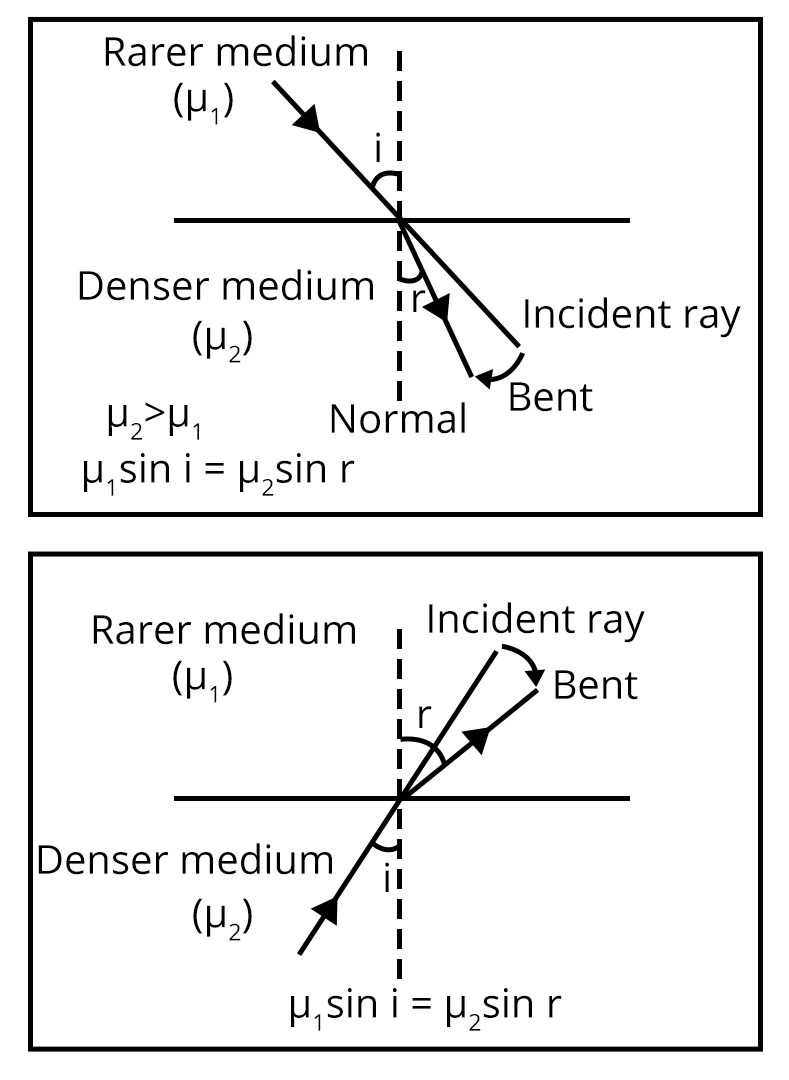5. Laws of refraction The ratio of angle of incidence (i) to the sign of angle of refraction (r) is a constant, which is known as the refractive index of second medium w.r.t. the first medium. $\dfrac{\sin i}{\sin r}= \dfrac{n_2}{n_1} = n_{21}$This is also called Snell’s law. Absolute refractive index (n) is defined as the ratio of speed of light in vacuum (c) to the speed of light in a medium (v).Therefore, $n = \dfrac{c}{v}$When the object is in the denser medium and observer is in the rarer medium, the relationship between apparent depth and real depth is given by$n_{12}= \dfrac{\text{Real depth}}{\text{Apparent depth}}$ 6. Power of a lens Power of a lens is the reciprocal of focal length of the lens. 7. Refraction from a Spherical Surface Let an object be placed in a medium with refractive index $n_{1}$ at distance u from the spherical surface. An image is in a medium with refractive index $n_{2}$ at a distance of v from the spherical surface. $\dfrac{n_2}{v}-\dfrac{n_1}{u} = \dfrac{n_2-n_1}{R}$ 8. Lens Lens is the refracting medium bound by 2 surfacesThe lens formula is given by:$\dfrac{1}{f}= \dfrac{1}{v}-\dfrac{1}{u}$Magnification of the object of height, h ket perpendicular to the principal axis and image height, h’ is given by:$m = \dfrac{h}{h'} =\dfrac{v}{u}= \dfrac{f}{f+u}=\dfrac{f-v}{f}$When the object is kept along the principal axis, the axial magnification (m’) is given by$m’= \lgroup\dfrac{v}{u}\rgroup^{2} = \lgroup\dfrac{f}{f+u}\rgroup^{2}= \lgroup\dfrac{f-v}{f}\rgroup^{2}$ 9. Total Internal Reflection When a light ray goes from denser medium to rarer medium, it bends away from the normal. For a certain angle of incidence i.e. angle of incidence ($i_{c}$) known as critical angle, the angle of refraction in the rarer medium becomes 90°. So, when the angle of incidence is greater than the critical angle, the light rays start reflecting back as shown in the figure below: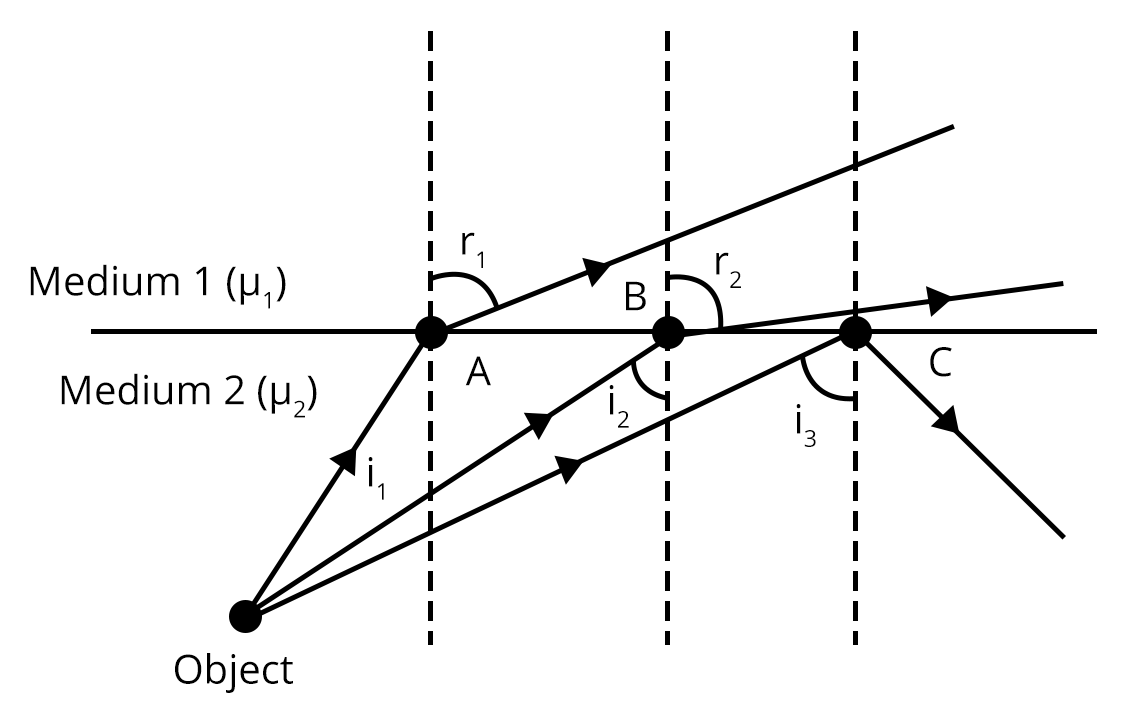10. Prism A prism is homogeneous, transparent medium bounded by two plane surfaces at an angles of A to each other 11. Scattering of light Molecules of medium after absorbing the incoming radiations emit the radiations in all the directions. This is called the scattering of light. 12. Optical Instruments An optical instrument is used to enhance and analyse the light waves 13. Simple Microscope It consists of a convex lens of small focal length and forms a magnified image when the object is placed between the optical centre and pole of the lens. 14. Compound Microscope It is formed using two convex lenses. One is known as the eyepiece and the other is called the objective lens. Focal length and aperture of the objective lens are smaller than that of the eyepiece. The image formed by the objective lens is real, inverted and magnified, which acts as the object for the eyepiece and the final image is highly magnified w.r.t the original object. 15. Telescope Telescope is an optical instrument which increases the visual angle of the eye by forming the image of distant objects at the least distance of distinct vision. 16. Resolving power of optical Instruments It is the ability of an optical instrument to produce distinct images of two points of an object placed very close together.

### List of Important Formulae

 SI. No Name of the Concept Formula 1. Mirror Formula $\dfrac{1}{f}= \dfrac{1}{v}+\dfrac{1}{u}$ 2. Magnification Produced by Spherical Mirrors $m = \dfrac{h}{h'} =-\dfrac{v}{u}= \dfrac{f}{f-u}=\dfrac{f-v}{f}$ 3. Axial Magnification Produced by Spherical Mirrors $m’= \lgroup\dfrac{v}{u}\rgroup^{2} = \lgroup\dfrac{f}{f-u}\rgroup^{2}= \lgroup\dfrac{f-v}{f}\rgroup^{2}$ 4. Snell’s Law $\dfrac{\sin i}{\sin r}= \dfrac{n_2}{n_1} = n_{21}$ 5. Power of Lens $P=\dfrac{1}{f}$ 6. Refraction from a Spherical Surface $\dfrac{n_2}{v}-\dfrac{n_1}{u} = \dfrac{n_2-n_1}{R}$ 7. Lens Formula $\dfrac{1}{f}= \dfrac{1}{v}-\dfrac{1}{u}$ 8. Magnification Produced by Lens $m = \dfrac{h}{h'} =\dfrac{v}{u}= \dfrac{f}{f+u}=\dfrac{f-v}{f}$ 9. Axial Magnification Produced by Lens $m’= \lgroup\dfrac{v}{u}\rgroup^{2} = \lgroup\dfrac{f}{f+u}\rgroup^{2}= \lgroup\dfrac{f-v}{f}\rgroup^{2}$ 10. Lens Maker’s Formula $P= \dfrac{1}{f} = (n_{21}-1)\lgroup\dfrac{1}{R_1}-\dfrac{1}{R_2}\rgroup$ 11. Total Internal Reflection $\sin i_c = \dfrac{1}{n_{12}}$ 12. Deviation Produced by Prism $\delta = i+e-A$For maximum deviation:$\mu= \dfrac{\sin\lgroup\dfrac{\delta_m+A}{2}\rgroup}{\sin\lgroup\dfrac{A}{2}\rgroup}$ 13. Magnification Produced by Simple Microscope $m= \lgroup1+\dfrac{D}{f}\rgroup$, where D = Normal viewing distance, f = focal length of the magnifying glass 14. Magnification Produced by Compound Microscope $m= -\dfrac{v_o}{u_o}\lgroup1+\dfrac{d}{f_o}\rgroup$ 15. Magnification Produced by Telescope $m = -\dfrac{f_o}{f_e}$ 16. Resolving Power of Optical Instruments RP = $\dfrac{1}{\text{d}\theta} = \dfrac{D}{1.22\lambda}$

### Solved Examples

1. A thin rod of length $\dfrac{f}{3}$ is lying along a principal axis of the concave mirror of focal length f. The image is real, magnified and inverted and one of the ends of the rod coincides with its image itself. Find the length of the image.

Sol:

The given image is real, magnified and inverted. So, the object must lie between the focus and centre of curvature of the concave mirror. Now, it is also given that the image of one of the ends of the rods coincides on itself which means that one end will be at the centre of curvature. The position of the rod must look like the figure shown below.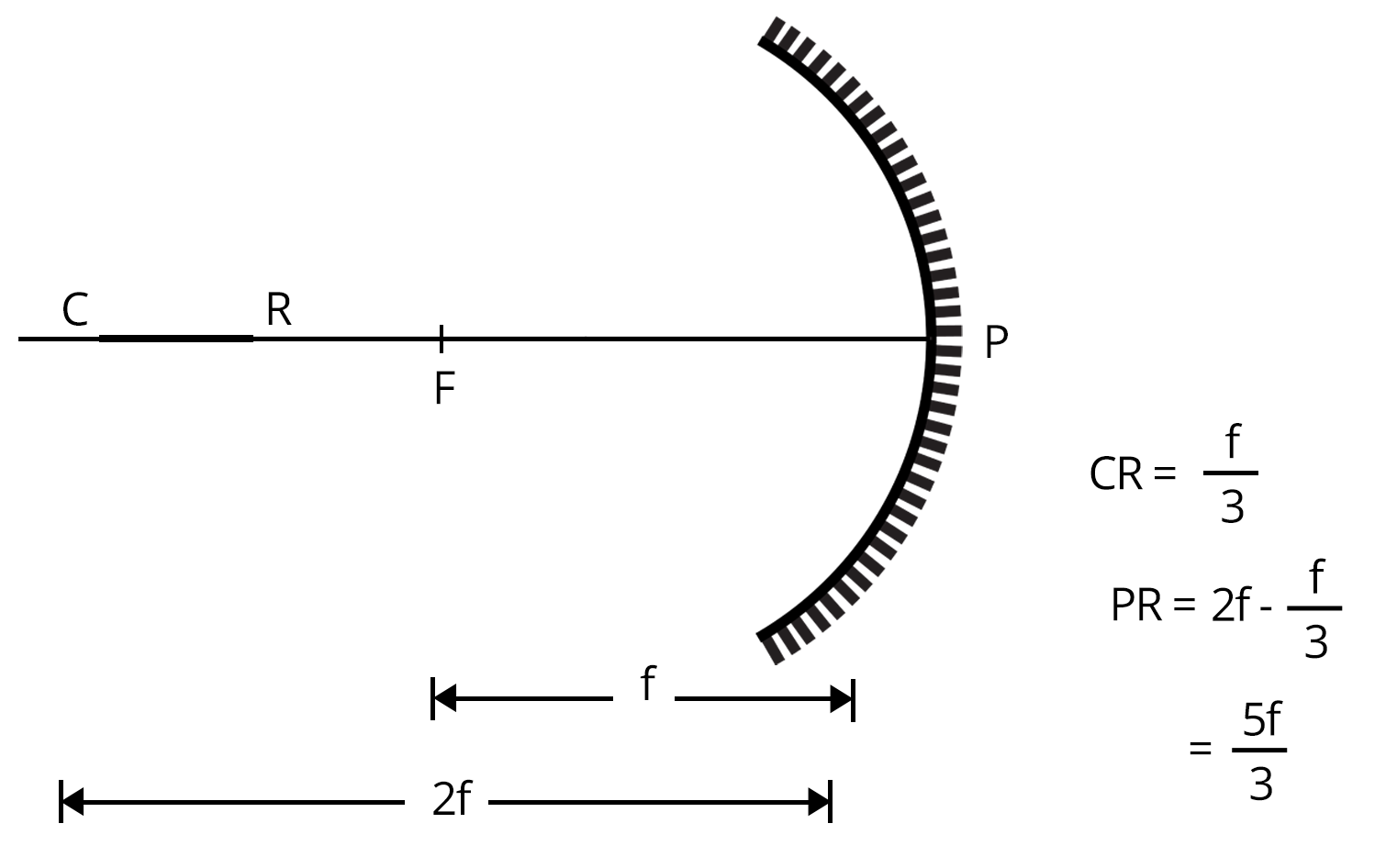The image of end C coincides with the end C of the object. Now, let us find the image distance of point R. The distance of R from the pole of the concave mirror is PR i.e. $\dfrac{5f}{3}$.

Therefore, object distance (u) = $-\dfrac{5f}{3}$,

Using mirror formula:

$\dfrac{1}{f}= \dfrac{1}{v}+\dfrac{1}{u}$

$-\dfrac{1}{f}= \dfrac{1}{v}-\dfrac{3}{5f}$

Solving the equation, we get

$v= -\dfrac{5f}{2}$

So, let the image of CR be C’R’ then it would look like the figure shown below: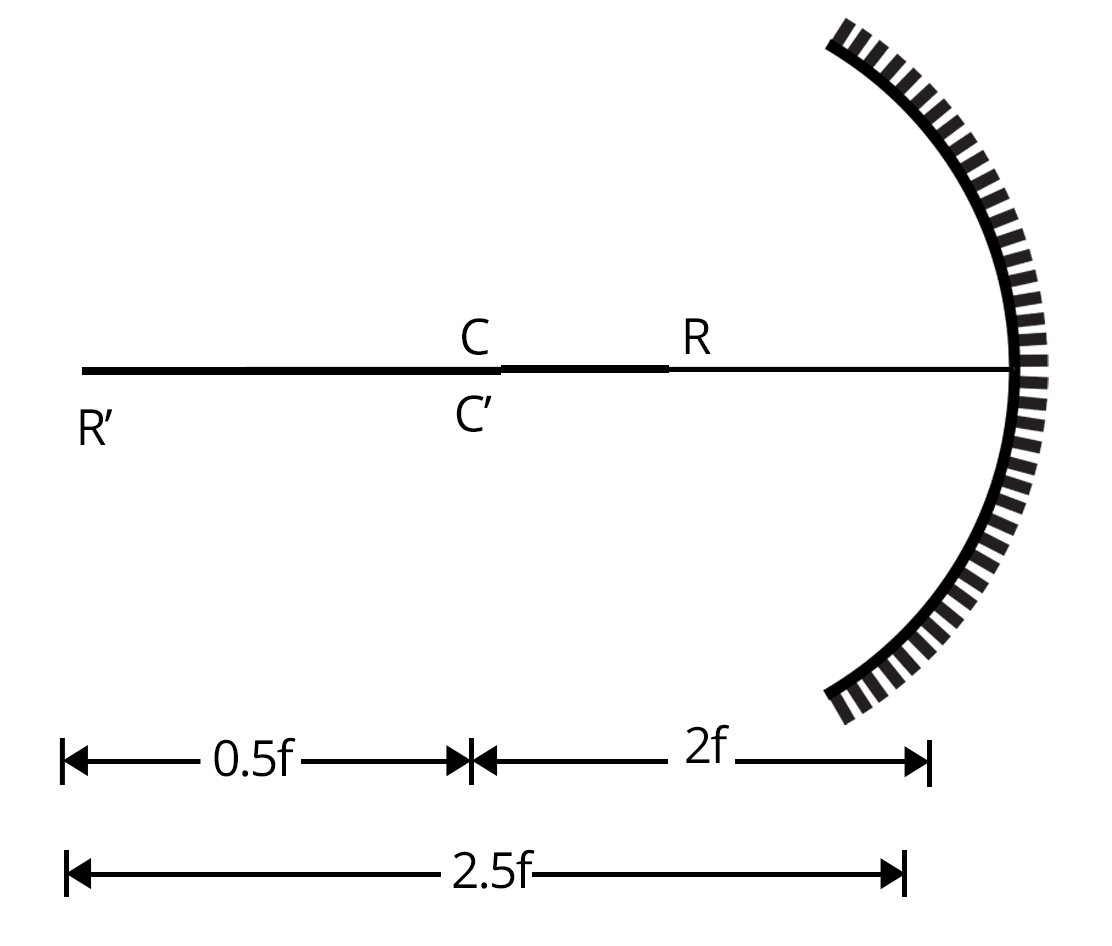From the figure, length of rod, CR will be 0.5f

Key point: In such a case, the magnification is given by m = $- \dfrac{\text{length of image}}{\text{length of object}}$

2. The angle of minimum deviation for a glass prism with $\mu= \sqrt{3}$ equals the refractive angle of the prism. What is the angle of the prism?

Sol:

Let,

The angle of the prism be A.

The angle of minimum deviation be $\delta_m$.

In the question, it is given that angle of minimum deviation is equal to the refractive angle of the prism, then A = $\delta_m$

Using the formula for minimum deviation:

$\mu=\dfrac{\sin\lgroup\dfrac{\delta_m+A}{2}\rgroup}{\sin\lgroup\dfrac{A}{2}\rgroup}$

Also given that $\mu= \sqrt{3}$

Therefore,

$\sqrt{3}= \dfrac{\sin\lgroup\dfrac{A+A}{2}\rgroup}{\sin\lgroup\dfrac{A}{2}\rgroup}$

$\sqrt{3}= \dfrac{\sin A}{\sin\lgroup\dfrac{A}{2}\rgroup}$

$\sqrt{3}=\dfrac{2\sin\lgroup\dfrac{A}{2}\rgroup\cos\lgroup\dfrac{A}{2}\rgroup}{\sin\lgroup\dfrac{A}{2}\rgroup}$

$\cos\lgroup\dfrac{A}{2}\rgroup= \dfrac{\sqrt{3}}{2}$

A = 60°

Therefore, the angle of the prism will be 60°.

Key Point: Minimum deviation occurs when the angle of incidence on the one plane of the prism is equal to the angle of emergence on the other plane of the prism.

### Previous Year Questions From NEET Paper

1. Find the value of the angle of emergence from the prism. The refractive index of the glass is $\sqrt{3}$     (NEET 2021)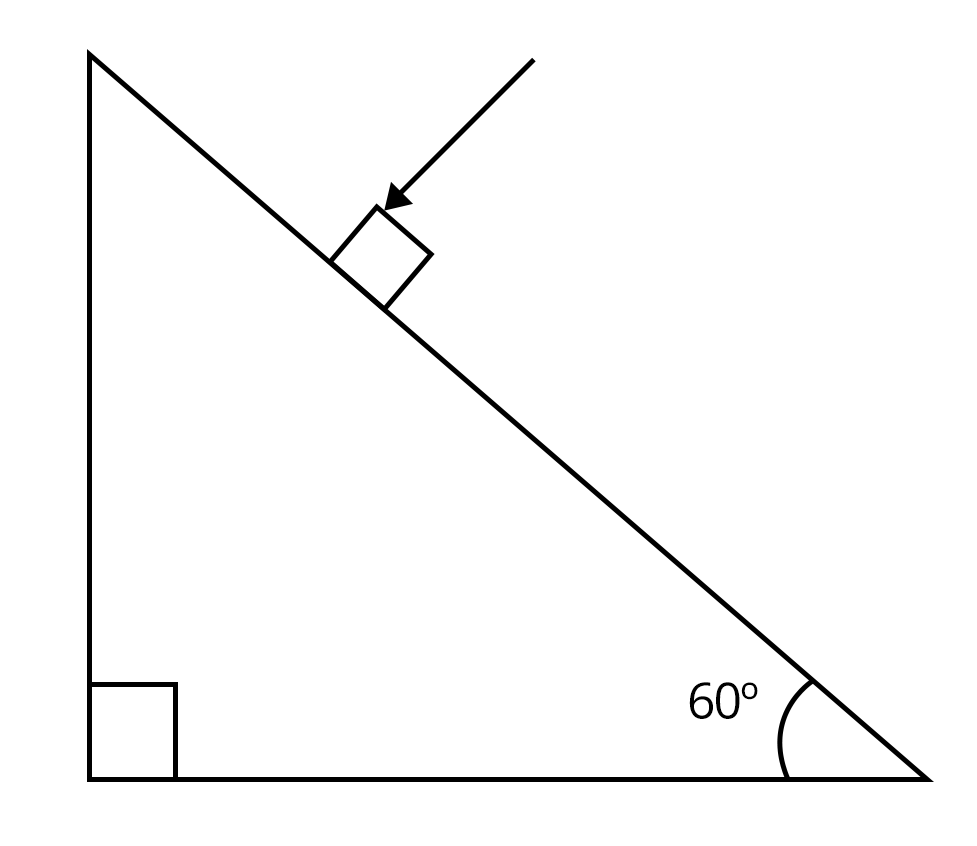1. 60°

2. 45°

3. 30°

4. 90°

Sol:

Given, the refractive index $(\mu_1) = \sqrt{3}$

We know, refractive index of air $(\mu_2) = 1$

Now, from the figure given below: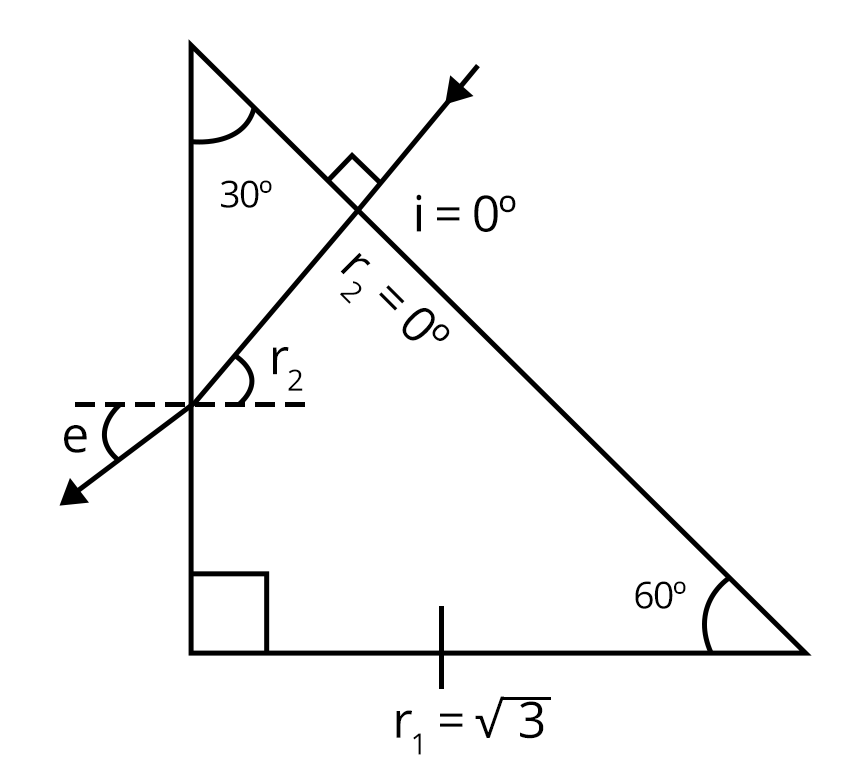Angle of refraction from the first surface, $r_1$= 0°

Also, $A = r_1+r_2$

Where, A = angle of prism

Therefore, 30°=0°+$r_2$

Then, $r_2$ = 30°

Now, using Snell’s law:

$\mu_2\sin\theta_2= \mu_1\sin\theta_1$

$\sqrt{3}\sin30= 1\times\sin e$

$\sin e = \dfrac{\sqrt{3}}{2}$

e = 60°

Hence, the correct answer is option (a).

Trick: In these types of questions, always complete the ray diagram first. It will help in understanding the situation well.

2. A lens of large focal length and large aperture is best suited as an objective of an astronomical telescope since (NEET 2021)

1. a large aperture contributes to the quality and visibility of the images.

2. a large area of the objective ensures better light-gathering power.

3. a large aperture provides a better resolution

4. All of the above.

Sol:

Resolving power of optical instruments is given by RP = $\dfrac{1}{\text{d}\theta} = \dfrac{D}{1.22\lambda}$. Therefore, the greater the aperture, the greater will be the resolving power and hence better resolution, and quality of the image will be formed.

Option (d) is the correct answer.

Trick: We use astronomical telescopes for better quality and resolution.

### Practice Questions

1. A ray of light is incident at an angle of 60° on the face of a prism having a refracting angle of 30°. The ray emerging out of the prism makes an angle of 30° with the incident ray. Show that the emergent ray is perpendicular to the face through which it emerges. (Ans. 0°)

2. A source of light is located at double focal length from a convergent lens. The focal length of the lens is f cm = 30. At what distance from the lens should a flat mirror be placed so that the rays reflected from the mirror are parallel after passing through the lens for the second time? (Ans. 45 cm)

### Conclusion

In this article, we have provided important information regarding the chapter Ray Optics and Optical Instruments such as important concepts, formulae, etc. Students should work on more solved examples for securing good grades in the NEET2022 exam.

See More## NEET Important Dates

View All Dates
NEET 2022 exam date and revised schedule have been announced by the NTA. NEET 2022 will now be conducted on 17-July-2022, and the exam registration closes on 20-May-2022. You can check the complete schedule on our site. Furthermore, you can check NEET 2022 dates for application, admit card, exam, answer key, result, counselling, etc along with other relevant information.
See More
View All Dates## NEET Information

Application Form
Eligibility Criteria
Reservation Policy
NTA has announced the NEET 2022 application form release date on the official website https://neet.nta.nic.in/. NEET 2022 Application Form is available on the official website for online registration. Besides NEET 2022 application form release date, learn about the application process, steps to fill the form, how to submit, exam date sheet etc online. Check our website for more details.## NEET 2022 Study Material

View NEET Syllabus in Detail
View NEET Syllabus in Detail## NEET 2022 Study Material

View all study material for NEET
All
Physics
Chemistry
Biology
See All## NEET Question Papers## NEET 2022 Book Solutions and PDF Download

View all NEET Important Books
Biology
NCERT Book for Class 12 Biology
Physics
NCERT Book for Class 12 Physics
Chemistry
NCERT Book for Class 12 Chemistry
Physics
H. C. Verma Solutions
See All## NEET Mock Tests

View all mock tests
"NEET 2022 free online mock test series for exam preparation are available on the Vedantu website for free download. Practising these mock test papers of Physics, Chemistry and Biology prepared by expert teachers at Vedantu will help you to boost your confidence to face the NEET 2022 examination without any worries. The NEET test series for Physics, Chemistry and Biology that is based on the latest syllabus of NEET and also the Previous Year Question Papers."
See More## NEET 2022 Cut Off

NEET Cut Off
NTA is responsible for the release of the NEET 2022 cut off score. The qualifying percentile score might remain the same for different categories. According to the latest trends, the expected cut off mark for NEET 2022 is 50% for general category candidates, 45% for physically challenged candidates, and 40% for candidates from reserved categories. For the general category, NEET qualifying marks for 2021 ranged from 720-138 general category, while for OBC/SC/ST categories, they ranged from 137-108 for OBC, 137-108 for SC and 137-108 for ST category.
See More## NEET 2022 Results

The NEET 2022 result is published by NTA on https://neet.nta.nic.in/ in the form of a scorecard on 7-Sep-2022. The scorecard will include the roll number, application number, candidate's personal details, and the percentile, marks, and rank of the candidate. Only those candidates who achieve the NEET cut-off will be considered qualified for the exam.
See More
Rank List
Counselling
Cutoff
NEET 2022 state rank lists will be released by the state counselling committees for admissions to the 85% state quota and to all seats in private medical and dental colleges. NEET 2022 state rank lists are based on the marks obtained in entrance exams. Candidates can check the NEET 2022 state rank list on the official website or on our site.## NEET Top Colleges

View all NEET 2022 Top Colleges
Want to know which Engineering colleges in India accept the NEET 2022 scores for admission to Engineering? Find the list of Engineering colleges accepting NEET scores in India, compiled by Vedantu. There are 1622 Colleges that are accepting NEET. Also find more details on Fees, Ranking, Admission, and Placement.
See More## FAQs on NEET Important Chapter - Ray Optics and Optical Instruments

FAQ

1. What is the weightage of the Ray Optics and Optical Instruments in NEET?

Nearly 3-4 questions arise in the exam from this chapter. Due to the weightage of the chapter, it is a must to practice this ch

2. What are the key points that need to be practised for solving questions from Ray Optics and Optical Instruments?

Students should concentrate on understanding the basics of reflection and refraction such as laws of refraction and reflection, rules for image formation in both reflection and refraction etc. and try solving different types of MCQ problems to grasp the concepts better. For NCERT, students should practice the derivations of important formulae.

3. Are previous year's questions enough for NEET?

To score 640+ in NEET, NCERT (both 11th and 12th) and previous year NEET Mains papers are sufficient. Solving previous 10-year NEET examinations offers us a tremendous advantage because 6-7 questions with identical alternatives are guaranteed to be repeated every year.## Notice board

News
BlogTrending pages